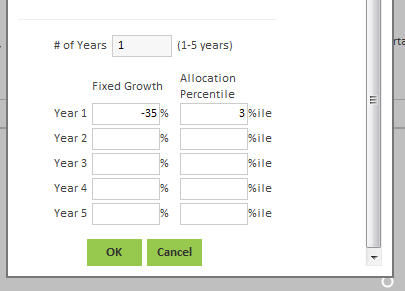# Major Loss, or Loss Capacity - What does 'allocation percentile' mean, and how does it correlate to market loss or gain? **Voyant Adviser, UK and Ireland**

Q - What is meant by 'allocation percentile' and how does this correlate to the actual percentage of market loss or gain?

A - It sounds that you're seeking to model a market crash, either by adding a 'Major Loss' event to your client's Timeline, or by using the software's 'Loss Capacity' simulation. It's quite likely that you have already clicked the (green) 'Event Settings', or 'Edit Settings' button, and are being presented with two columns - one headed 'Fixed Growth', the other headed 'Allocation Percentile', as shown below:Note that there are two ways to grow the value of accounts, within Voyant, either by using simple 'fixed growth' rates, or by applying an asset allocation (relying on assumptions about each of the individual asset classes therein). These options are not mutually exclusive, and one can easily combine both approaches, within the same client plan. What's important to understand is that: how the market crash is applied (by the software) will be different for accounts using a 'fixed growth' rate, compared with accounts using an asset allocation.

If all of the accounts within your client plan are operating solely on 'fixed growth' rates, then one can simply ignore the column labelled 'Allocation Percentile', as this won't be relevant. The 'Allocation Percentile' column is only applicable to accounts being grown by way of an asset allocation. The importance of 'allocation percentile' is that this is a relative (and probabilistic) measure of performance*, rather than an absolute measure - the consequence is that one should expect to get a nuanced response to the assumed market crash, dependent upon the underlying asset allocation of one's accounts, e.g. an account allocated entirely to domestic fixed interest, will not behave/respond to the crash in the same way as an account allocated entirely to overseas equity. It follows from this, of course, that there is not a straightforward correlation between the 'allocation percentile' value entered, and the resulting rate of return.

*To emphasize, the 'percentile' values, used in the software's market-based simulations, are a measure of relative performance (i.e. relative to the assumed mean). The resulting output will be determined by one's underlying market assumptions (mean and standard deviation values, as located under Preferences > Market Assumptions). The 'allocation percentile' value that is entered will be applied to any accounts in your plan that are set to be grown using an asset allocation, rather than a 'fixed growth' rate.

Q - When I enter/change the value under 'Fixed Growth', the corresponding value under 'Allocation Percentile' changes - why is this?

Firstly, note that one can always overwrite the existing (default) value shown under either 'Fixed Growth', or 'Allocation Percentile', meaning they can both be set to any value desired. It is the case, nonetheless - when one enters a value under 'Fixed Growth' - that the software will interpret this value for the purpose of any accounts (in your client plan) that happen to be utilising an asset allocation. The software will do this by taking the 'Fixed Growth' value you have entered, and comparing it to returns (mean, and standard deviation values) for the FTSE All Share between 1900 and 2017, to arrive at a measure of relative performance, given the assumption that investment returns are normally distributed. The assumption of normal distribution means that returns conform to a bell curve probability distribution. For further reading, one might refer to Wikipedia, for example: http://en.wikipedia.org/wiki/Normal_distribution

The assumption of normal distribution enables the software to take a given 'fixed growth' return (e.g. -30%), and determine the relative probability of such an outcome, historically speaking. This value is called the Z-score and it represents the number of standard deviations away from (above, or below) the mean. If the Z-score is 1, for example, then the investment return value is 1 standard deviation above the mean, which corresponds (approximately) to the 84th percentile. Returning to the example above - i.e. a return of -30% - the FTSE All Share dataset gives us a Z-score of -1.72, which translates to an allocation percentile value of approximately 4. One can interpret this value - in turn - as meaning that the annual return of the FTSE All Share (between 1900 and 2010) will have improved upon -30% approximately 96% of the time.

To reiterate, one does not need to run with the software's suggested 'allocation percentile' value(s) - its calculated percentile values are merely a suggestion, based on the 'fixed growth' value(s) entered by the user. As stated, one can overwrite the suggested 'allocation percentile' to any value between 0 and 100.